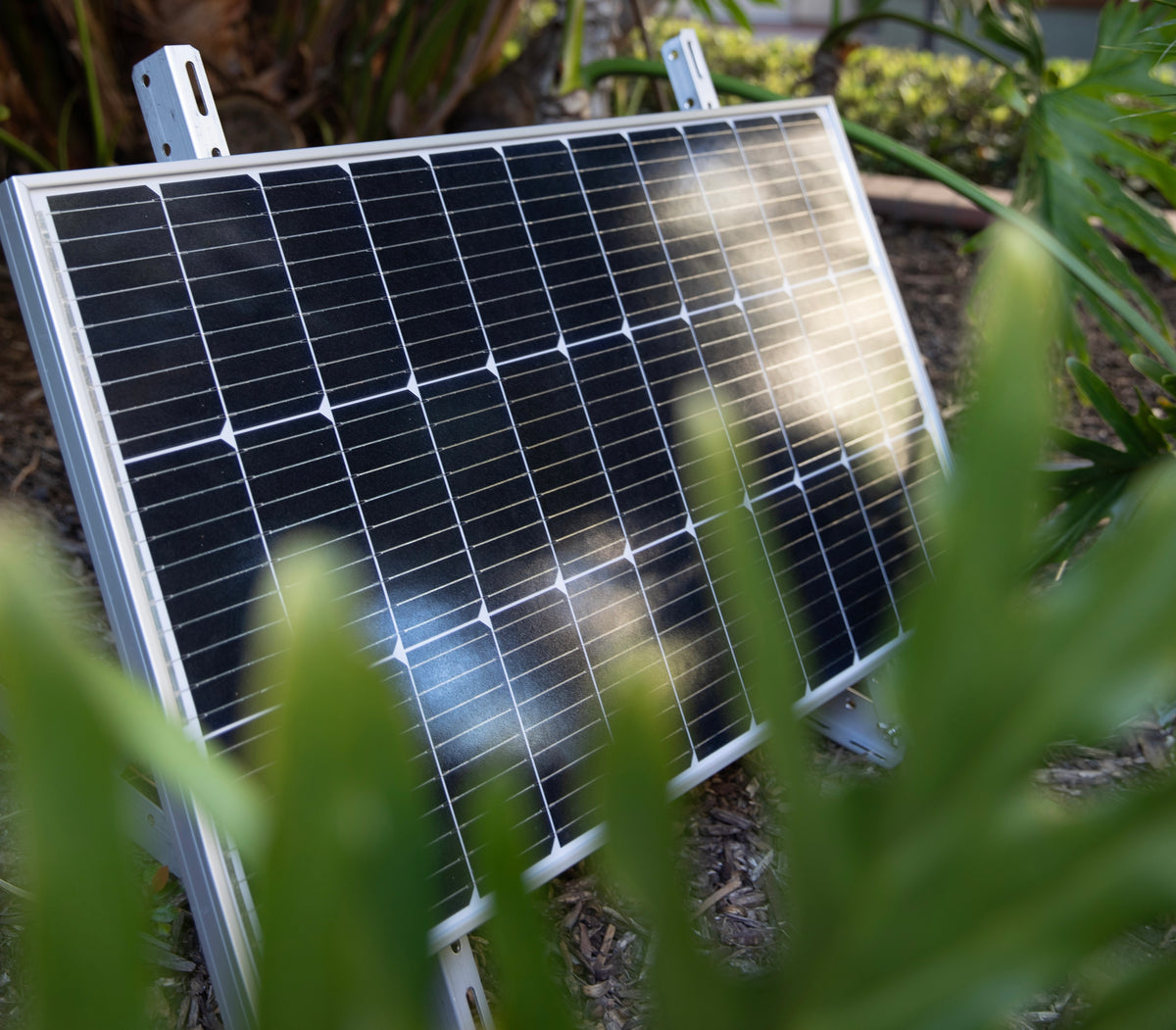# What size of solar panel will charge a 400 Ah battery?

Solar panels and batteries are two key components of an off-grid solar power system. The size of the solar panel required to charge a 400 Ah battery depends on several factors, such as the capacity of the solar panel, the efficiency of the panel, the weather conditions, and the amount of sunlight received.

A 400 Ah battery is a large capacity battery and would require a significant amount of power to be charged fully. The power required to charge the battery would depend on the depth of discharge, or how much of the battery's capacity has been used. For example, if the battery is discharged to 50%, it would require twice the amount of power to fully recharge it compared to if it was only discharged to 25%.

To determine the size of the solar panel required to charge a 400 Ah battery, you need to calculate the amount of energy the battery can store and the amount of energy the solar panel can produce.

Let's assume that the battery is a 12V battery, and the minimum voltage required to charge the battery is 14.4V. This means that the battery requires a charging voltage of 14.4V or higher to charge correctly.

To charge a 400 Ah battery, we need to know the amount of energy required to charge the battery from its current state to its fully charged state. This can be calculated using the following formula:

Energy (Wh) = Battery Voltage (V) x Battery Capacity (Ah) x Depth of Discharge (%)

Using a 12V, 400 Ah battery with a depth of discharge of 50%, the energy required to charge the battery would be:

Energy (Wh) = 12V x 400 Ah x 50% = 2,400 Wh

Now we need to calculate the amount of power the solar panel can produce. The power output of a solar panel depends on several factors such as the panel's size, efficiency, and the amount of sunlight received.

Assuming that the solar panel has an efficiency of 20% and receives 5 hours of sunlight per day, the power output can be calculated using the following formula:

Power (W) = Solar Panel Size (m2) x Solar Irradiance (W/m2) x Efficiency (%)

Let's assume that the solar panel size is 10 m2, and the solar irradiance is 1,000 W/m2. The power output of the solar panel would be:

Power (W) = 10 m2 x 1,000 W/m2 x 20% = 2,000 W

To determine the size of the solar panel required to charge the 400 Ah battery, we need to divide the energy required to charge the battery by the power output of the solar panel.

Solar Panel Size (W) = Energy (Wh) / (Solar Panel Size (m2) x Solar Irradiance (W/m2) x Efficiency (%))

Using the values calculated above, the size of the solar panel required to charge a 400 Ah battery would be:

Solar Panel Size (W) = 2,400 Wh / (10 m2 x 1,000 W/m2 x 20%) = 1,200 W

In summary, to charge a 400 Ah battery with a depth of discharge of 50%, a solar panel with a power output of at least 1,200 W would be required. However, it's essential to note that the actual size of the solar panel required would depend on several factors, such as weather conditions, the efficiency of the panel, and the amount of sunlight received.